# Split Violin Plots

#### 2019-11-29

##Violin Plots

Therefore violin plots are a powerful tool to assist researchers to visualise data, particularly in the quality checking and exploratory parts of an analysis. Violin plots have many benefits:

• Greater flexibility for plotting variation than boxplots
• More familiarity to boxplot users than density plots
• Easier to directly compare data types than existing plots

As shown below for the iris dataset, violin plots show distribution information that the boxplot is unable to.

###General Set up

library("vioplot")

We set up the data with two categories (Sepal Width) as follows:

data(iris)
summary(iris$Sepal.Width) ## Min. 1st Qu. Median Mean 3rd Qu. Max. ## 2.000 2.800 3.000 3.057 3.300 4.400 table(iris$Sepal.Width > mean(iris$Sepal.Width)) ## ## FALSE TRUE ## 83 67 iris_large <- iris[iris$Sepal.Width > mean(iris$Sepal.Width), ] iris_small <- iris[iris$Sepal.Width <= mean(iris$Sepal.Width), ] ###Boxplots First we plot Sepal Length on its own: boxplot(Sepal.Length~Species, data=iris, col="grey")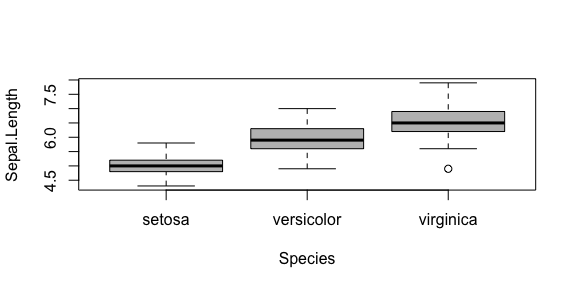An indirect comparison can be achieved with par: { par(mfrow=c(2,1)) boxplot(Sepal.Length~Species, data=iris_small, col = "lightblue") boxplot(Sepal.Length~Species, data=iris_large, col = "palevioletred") par(mfrow=c(1,1)) }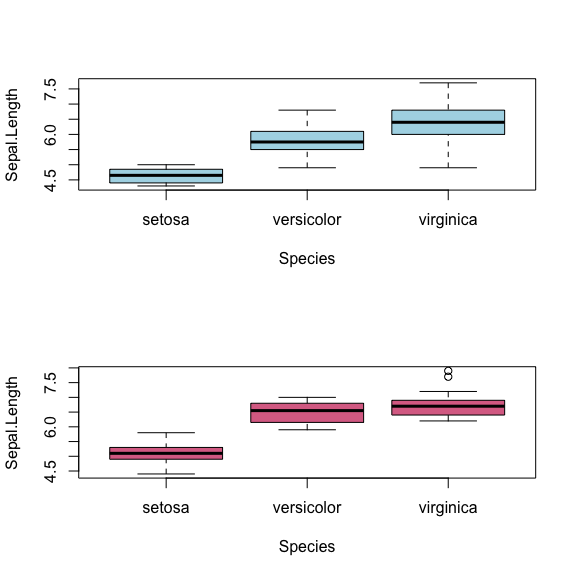### Violin Plots First we plot Sepal Length on its own: vioplot(Sepal.Length~Species, data=iris)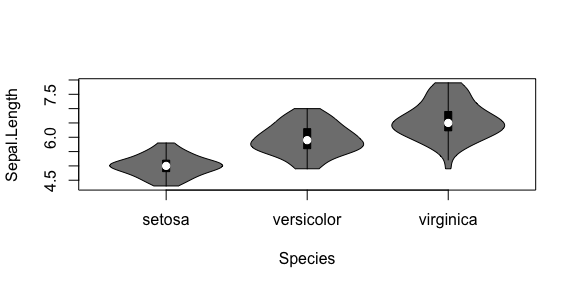An indirect comparison can be achieved with par: { par(mfrow=c(2,1)) vioplot(Sepal.Length~Species, data=iris_small, col = "lightblue", plotCentre = "line") vioplot(Sepal.Length~Species, data=iris_large, col = "palevioletred", plotCentre = "line") par(mfrow=c(1,1)) }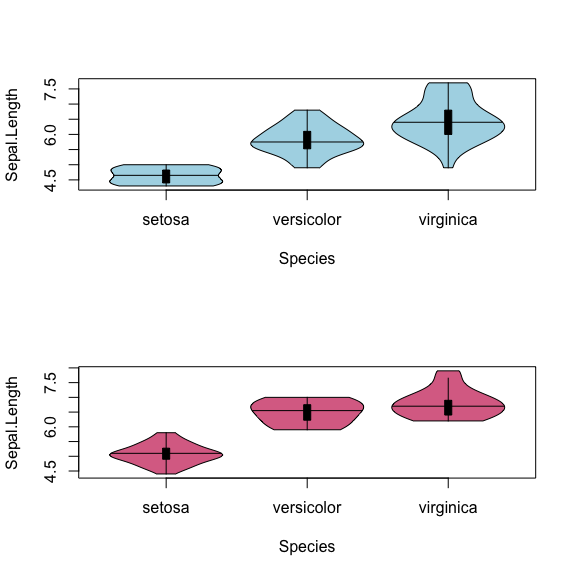### Split Violin Plots A more direct comparision can be made with the side argument and add = TRUE on the second plot: vioplot(Sepal.Length~Species, data=iris_large, col = "palevioletred", plotCentre = "line", side = "right") vioplot(Sepal.Length~Species, data=iris_small, col = "lightblue", plotCentre = "line", side = "left", add = T) title(xlab = "Species", ylab = "Sepal Length") legend("topleft", fill = c("lightblue", "palevioletred"), legend = c("small", "large"), title = "Sepal Width")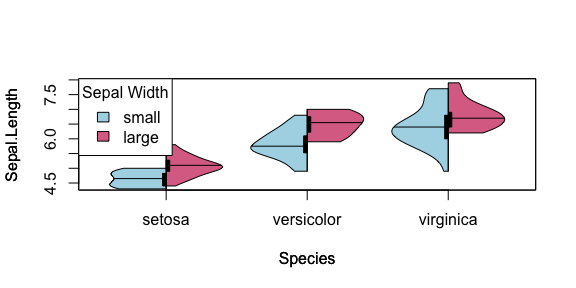### median The line median option is more suitable for side by side comparisions but the point option is still available also: vioplot(Sepal.Length~Species, data=iris_large, col = "palevioletred", plotCentre = "point", side = "right", pchMed = 21, colMed = "palevioletred4", colMed2 = "palevioletred2") vioplot(Sepal.Length~Species, data=iris_small, col = "lightblue", plotCentre = "point", side = "left", pchMed = 21, colMed = "lightblue4", colMed2 = "lightblue2", add = T) title(xlab = "Species", ylab = "Sepal Length") legend("topleft", fill = c("lightblue", "palevioletred"), legend = c("small", "large"), title = "Sepal Width")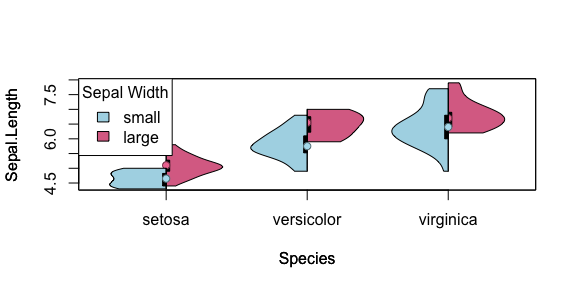It may be necessary to include a points command to fix the median being overwritten by the following plots: vioplot(Sepal.Length~Species, data=iris_large, col = "palevioletred", plotCentre = "point", side = "right", pchMed = 21, colMed = "palevioletred4", colMed2 = "palevioletred2") vioplot(Sepal.Length~Species, data=iris_small, col = "lightblue", plotCentre = "point", side = "left", pchMed = 21, colMed = "lightblue4", colMed2 = "lightblue2", add = T) points(1:length(levels(iris$Species)), as.numeric(sapply(levels(iris$Species), function(species) median(iris_large[grep(species, iris_large$Species),]$Sepal.Length))), pch = 21, col = "palevioletred4", bg = "palevioletred2") title(xlab = "Species", ylab = "Sepal Length") legend("topleft", fill = c("lightblue", "palevioletred"), legend = c("small", "large"), title = "Sepal Width")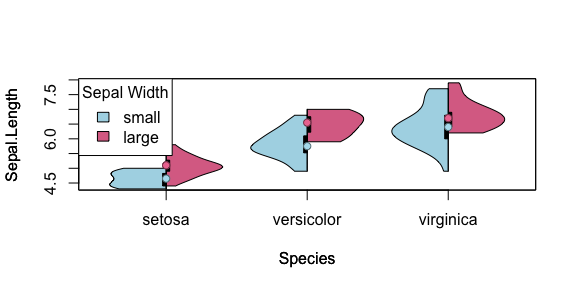Similarly points could be added where a line has been used previously: vioplot(Sepal.Length~Species, data=iris_large, col = "palevioletred", plotCentre = "line", side = "right", pchMed = 21, colMed = "palevioletred4", colMed2 = "palevioletred2") vioplot(Sepal.Length~Species, data=iris_small, col = "lightblue", plotCentre = "line", side = "left", pchMed = 21, colMed = "lightblue4", colMed2 = "lightblue2", add = T) points(1:length(levels(iris$Species)), as.numeric(sapply(levels(iris$Species), function(species) median(iris_large[grep(species, iris_large$Species),]$Sepal.Length))), pch = 21, col = "palevioletred4", bg = "palevioletred2") points(1:length(levels(iris$Species)), as.numeric(sapply(levels(iris$Species), function(species) median(iris_small[grep(species, iris_small$Species),]\$Sepal.Length))), pch = 21, col = "lightblue4", bg = "lightblue2")
title(xlab = "Species", ylab = "Sepal Length")
legend("topleft", fill = c("lightblue", "palevioletred"), legend = c("small", "large"), title = "Sepal Width")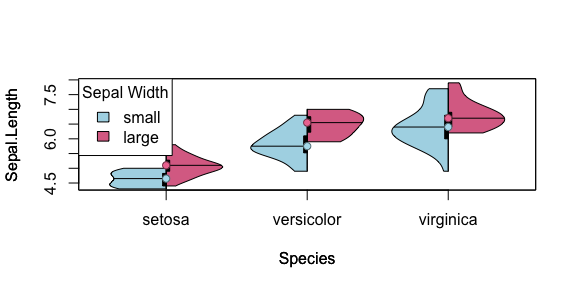Here it is aesthetically pleasing and intuitive to interpret categorical differences in mean and variation in a continuous variable.

#### Sources

These extensions to vioplot here are based on those provided here:

These have previously been discussed on the following sites: